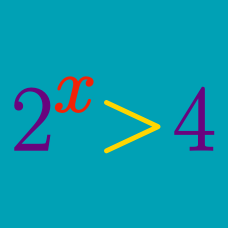Algebra

# Exponential Inequalities: Level 2 Challenges

$\Large \color{#3D99F6}{71^{70}} \quad \text{or} \quad \color{#D61F06}{ 70^{71} }$

Which one of the two numbers above is greater?

True or False?

$\quad$ If $\color{#D61F06}{a},\color{#3D99F6}{b},$ and $\color{#20A900}{c}$ are non-zero reals and $\color{#D61F06}{a}>\color{#3D99F6}{b},$ then $\color{#D61F06}{a}^{\color{#20A900}{c}} > \color{#3D99F6}{b}^{\color{#20A900}{c}}.$

How many positive integers $n$ are there such that $10^n \leq n^{10}$?

$\large |x|^{(x^2-x-2)} < 1$

If the solution to the inequality above is $x\in (A,B)$, then find the value of $A+B$.

Given three numbers such that $0 < a < b < c < 1$, define

$A = a^{a}b^{b}c^{c}, \quad B = a^{a}b^{c}c^{b} , \quad C = a^{b}b^{c}c^{a}.$

How do the values of $A, B, C$ compare to each other?

×